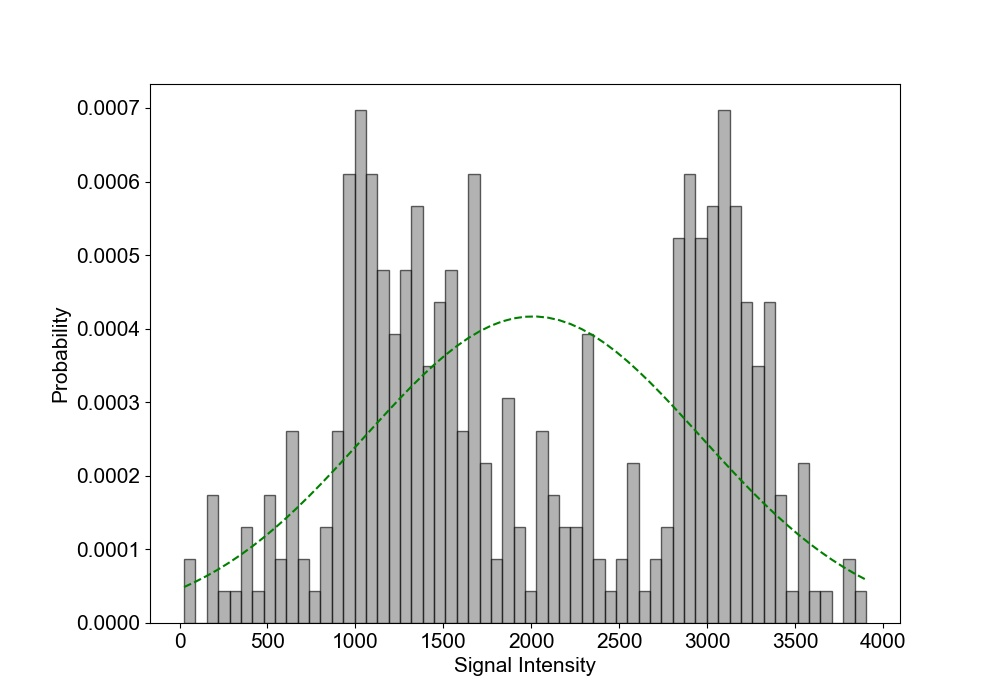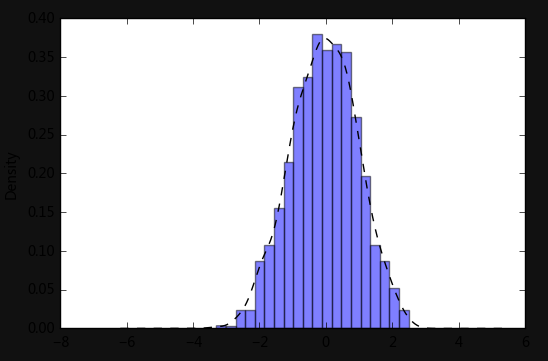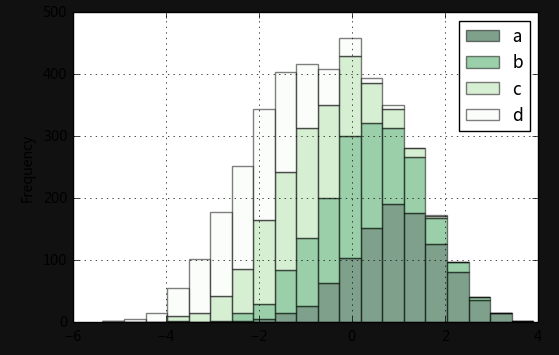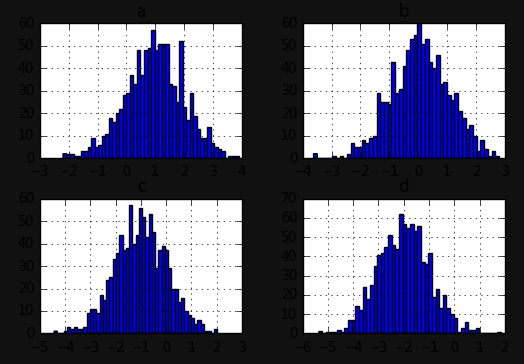• 在python中一般采用matplotlib库的hist来绘制直方图，至于如何给直方图添加拟合曲线（密度函数曲线），一般来说有以下两种方法。 方法一：采用matplotlib中的mlab模块 mlab模块是Python中强大的3D作图工具，立体感...
• 使用Matplotlib，基于频率分布直方图，为之添加概率密度曲线和数学表达式。

绘制目标

我们可以单独使用直方图来描述定量数据的分布特征，而如果为直方图添加一条概率密度曲线，就能更明显地去观察这种特征。

Note：不熟悉正态分布、概率密度、频率分布直方图等内容的麻烦复习一下概率论的内容。

Matplotlib编程实现

# -*- coding:utf-8 -*-

import numpy as np
import matplotlib.pyplot as plt
import matplotlib as mpl

mpl.rcParams["font.sans-serif"] = ["KaiTi"]
mpl.rcParams["axes.unicode_minus"] = False

mu = 60.0
sigma = 2.0
x = mu + sigma*np.random.randn(500)

bins = 50

fig, ax = plt.subplots(1, 1)

n, bins, patches = ax.hist(x, bins, density=True, histtype="bar", facecolor="#99FF33", edgecolor="#00FF99", alpha=0.75)

y = ((1/(np.power(2*np.pi, 0.5)*sigma))*np.exp(-0.5*np.power((bins-mu)/sigma, 2)))

ax.plot(bins, y, color="#7744FF", ls="--", lw=2)

ax.grid(ls=":", lw=1, color="gray", alpha=0.2)

ax.text(54, 0.2, r"$y=\frac{1}{\sqrt{2\pi}\sigma}e^{-\frac{(x-\mu)^2}{2\sigma^2}}$", {"color":"#FF5511", "fontsize":20})

ax.set_xlabel("体重")
ax.set_ylabel("概率密度")
ax.set_title(r"体重的直方图：$\mu=60.0$, $\sigma=2.0$", fontsize=16)

plt.show()

成品图展开全文• 首先，还是看看matplotlib中hist函数的语法 hist( x, bins=None, range=None, normed=False, # 超重要 density=False, weights=None, cumulative=False, bottom=None, histtype='bar', align='mid', rwidth=None...

首先，还是看看matplotlib中hist函数的语法

hist( x, bins=None, range=None, normed=False, # 超重要
density=False, weights=None, cumulative=False, bottom=None, histtype='bar', align='mid', rwidth=None, # 选用
orientation='vertical', color=None, edgecolor=None, label=None, # 常用
stacked=False, **kwargs)

x：数组或者数组序列（不要求每个数组长度相同），用于存放数据；
bins：整数、序列或字符串，整数表示等宽区间的个数（直方图条形个数），自动计算区间范围，序列则表示区间的范围，除了最后一个以外，都是包含左边不包含右边，字符串则表示对应的策略，默认为 hist.bins；
range：元组，指定最小值和最大值（直方图数据的上下界），默认包含绘图数据中的最小值和最大值（默认以绘图数据的最小值作为直方图数据的上下界）；
normed：是否将直方图的频数转化为频率；
density：布尔值，可选，如果为 True，则返回的是归一化的概率密度，所有区间的概率之和为1；
weights：类似于数组的值，可选，形状和 x xx 相同，表示每个值对应的权重，默认情况下所有数据的权重相同；
cumulative：布尔值或 -1，累积求和，表示小于某个数的所有元素个数之和，随着数的增大而不断增大，如果为 -1，则反转方向，表示大于某个数的所有元素个数之和，随着数量增大而不断减小；
histtype：指定直方图的类型，默认为 bar。bar（多个并列摆放），barstacked（多个堆叠摆放），step（生成对应的折线），stepfilled（填充相关区域）；
align：设置条形边界值的对齐方式，默认为 mid，另外还有 left 和 right；
rwidth：设置条形图的宽度；
orientation：设置直方图的摆放方向，默认为垂直方向；
color：设置直方图的填充色；
edgecolor：设置直方图边框色；
label：设置直方图标签，可以通过 legend 展示其图例；
stacked：当有多个数据时，是否需要将直方图呈堆叠摆放，默认水平摆放

示例：

import numpy as np
import matplotlib.pyplot as plt
from scipy.stats import norm

plt.rcParams['font.family']='Arial' # 设置字体和figure大小
plt.rcParams['font.size']=15
plt.figure(figsize=(10,7))
#
mu =np.mean(data) #计算均值
sigma =np.std(data) #计算标准差

num_bins = 60 #直方图柱子的数量

n, bins, patches = plt.hist(data, num_bins,density=True, edgecolor="black",facecolor='gray', alpha=0.6,range=[int(tumor_min),int(tumor_max)])
#直方图函数，x为x轴的值，normed=1表示为概率密度，即和为一，绿色方块，色深参数0.5.返回n个概率，直方块左边线的x值，及各个方块对象
y = norm.pdf(bins, mu, sigma) #拟合一条最佳正态分布曲线y
plt.plot(bins, y, 'g--') #绘制y的曲线
plt.xlabel('Signal Intensity') #绘制x轴
plt.ylabel('Probability') #绘制y轴
plt.show()

效果：展开全文python
• matplotlib中，ax.plot函数是用来画曲线的，而ax.hist函数则用来画直方图（histogram）。下面我们用python代码换一个直方图，10000个符合正态分布的随机数，将他们按照直方图画出来：import numpy as npimport ...

在matplotlib中，ax.plot函数是用来画曲线的，而ax.hist函数则用来画直方图（histogram）。

下面我们用python代码换一个直方图，10000个符合正态分布的随机数，将他们按照直方图画出来：

import numpy as np

import matplotlib.pyplot as plt

x = np.random.randn(10000)

fig = plt.figure()

ax.hist(x, bins=50, color='blue', alpha=0.7)

plt.show()

注意ax.hist函数，数据的输入只有x，x可以是一个sequence，也可以是matrix；

bins控制直方图中等宽分组的数量，默认为10，不过应该很少用默认值吧；

alpha控制直方图颜色的透明度。（plot等其它函数中，也有这个参数）正态分布直方图

这个直方图怎么看，y轴表示数据出现的频率或次数，x轴是按bins参数输入进行分组。直方图，可以理解为由一系列高度不等的纵向条柱来表示数据分布特征的统计报告图，它是对原始数据进行压缩的结果。

直方图分组的区间

我没有找到特别权威的说明，不过，通过阅读matplotlib官方教材能够发现，bins的值如果是一个整数，x轴的区间就进行等宽划分；bins只也可以是一个sequence对象，用来进行不等宽划分x轴。

不管怎么分x轴的区间，每一个分组，都是左封闭右开放，只有最后一个分组，是左右都封闭的！（想一想这样是合理的，左边不封闭，最左边的那个分组就没法处理了）

>>> plt.hist((1,2,3,4,5,6,7,8,2), bins=(1,2,3,4,5,6,8))

(array([1., 2., 1., 1., 1., 3.]), array([1, 2, 3, 4, 5, 6, 8]),

ject of 6 artists>)

对(1,2,3,4,5,6,7,8,2)话直方图，但是bins=(1,2,3,4,5,6,8)，即6-8为一个大分组，有3个数字落在这个区间，数据中出现了两次2，第1个分组是[1,2)，不包含2，2因此包含在第2个分组中[2,3)。这样画出来的直方图如下：bins实现非等宽划分组

上面这个图其实看着不是很好看，全都粘在一起了，我们可以通过rwidth参数（float，分组区间大小的百分比）来控制各个bar之间的间隔，注意此时图像上的位置，已经不再是真实的分组区间，x轴的刻度也可以用xticks函数来精确控制：

>>> plt.hist((1,2,3,4,5,6,7,8,2), bins=(1,2,3,4,5,6,8), rwidth=0.9, color='purpl

e', alpha=0.5)

(array([1., 2., 1., 1., 1., 3.]), array([1, 2, 3, 4, 5, 6, 8]),

ject of 6 artists>)

>>> plt.xticks(range(1,10,1))

效果如下：美化后的直方图

当bins参数为一个sequence时，实际上就是给dataset指定了一个range，即bins所括起来的区间不需要与dataset的区间一致。当bins是一个int时，我们可以用range参数来指定有效区间，将不在区间范围内的outliers排除在直方图的统计中。

输入数据为matrix时的直方图

输入数据x如果是一个matrix，matplotlib会把它的每一个列向量当做一个dataset来画直方图，然后将多个直方图并排在一起放在一个图中。此时，分组在x轴上的分割就不是精确的了（就像使用了rwidth参数）：

>>> plt.hist(((1,2,3,4,5,1,2,3,4,5,1,1,2,2,3,4,5,5),(1,2,3,4,5),(1,2,3,3,3)), bi

ns=4)

(array([[4., 4., 3., 7.],

[1., 1., 1., 2.],

[1., 1., 3., 0.]]), array([1., 2., 3., 4., 5.]),

r objects>)

从返回值可以看出，分组为array([1., 2., 3., 4., 5.])，但为了作图，多个柱形公用一个区间，他们只能在这个区间内进行某种排列，两边还要留点空余出来：多个dataset时的直方图

根据需要，我们可以此时将多个直方图的bar堆叠起来（stack），使用stacked=True参数，配合rwidth，代码和效果如下：

>>> plt.hist(((1,2,3,4,5,1,2,3,4,5,1,1,2,2,3,4,5,5),(1,2,3,4,5),(1,2,3,3,3)), bi

ns=4, stacked=True, rwidth=0.9)

(array([[4., 4., 3., 7.],

[5., 5., 4., 9.],

[6., 6., 7., 9.]]), array([1., 2., 3., 4., 5.]),

展开全文• 直方图+密度图2.堆叠直方图 plt.hist(x, bins=10, range=None, normed=False, weights=None, cumulative=False, bottom=None, histtype='bar', align='mid', orientation='vertical',rwidth=None, log=False, color...

文章目录

plt.hist(x, bins=10, range=None, normed=False, weights=None, cumulative=False, bottom=None,
histtype='bar', align='mid', orientation='vertical',rwidth=None, log=False, color=None, label=None,
stacked=False, hold=None, data=None, **kwargs)

1.直方图+密度图

# 直方图+密度图

s = pd.Series(np.random.randn(1000))
s.hist(bins = 20,
histtype = 'bar',
align = 'mid',
orientation = 'vertical',
alpha=0.5,
normed =True)
# bin：箱子的个数
# normed 标准化(将值放在[0,1]区间内)
# histtype 风格，bar，barstacked（堆叠），step（外轮廓），stepfilled（外轮廓+填充）
# orientation 水平还是垂直{‘horizontal’, ‘vertical’}
# align : {‘left’, ‘mid’, ‘right’}, optional(对齐方式)

s.plot(kind='kde',style='k--')
# 密度图
# 密度图只显示在（0，1）区间内，因此叠加的时候直方图需要标准化！2.堆叠直方图

# 堆叠直方图

plt.figure(num=1)
df = pd.DataFrame({'a': np.random.randn(1000) + 1, 'b': np.random.randn(1000),
'c': np.random.randn(1000) - 1, 'd': np.random.randn(1000)-2},
columns=['a', 'b', 'c','d'])
df.plot.hist(stacked=True,
bins=20,
colormap='Greens_r',
alpha=0.5,
grid=True)
# 使用DataFrame.plot.hist()和Series.plot.hist()方法绘制
# stacked：是否堆叠

df.hist(bins=50)
# 生成多个直方图展开全文python
• 1、直方图(Histograms)直方图是显示频率分布的图。该图显示了每个给定间隔内的观察次数。示例：假设您要求250人的身高，则最终可能会得到如下所示的直方图：可以从直方图中看到大约有：2人从140到145厘米5人从145到...
• 直方图与柱状图外观表现很相似，用来展现连续型数据分布特征的统计图形(柱状图主要展现离散型数据分布)，官方hist项目地址。函数：matplotlib.pyplot.hist(x,bins=None,range=None, density=None, bottom=None, ...
• 这就是为什么我要对拟合曲线和相关的直方图使用相同的颜色，以使其具有一定的可区分性。我找不到任何与此相关的信息。我找到了一个解决方案plt.gca().set_color_cycle(None)感谢在Matplotlib中重置颜色周期下面的...
• 说明:本程序参考刘顺祥老师的<...我们可以使用直方图来展现数据的分布，同过图形的长相，就可以快速的判断数据是否近似服从正态分布。之所以我们很关心数据的分布，是因为在统计学中，很多假设条件都会...
• python之matplotlib.pyplot直方图

万次阅读 2018-08-23 18:04:57
反正画直方图比折线图在EXCEL上方便太多了。 直方图介绍 直方图参数说明 官网案例 实际案例 直方图介绍 直方图(Histogram)又称质量分布图。是一种统计报告图，由一系列高度不等的纵向条纹或线段表示数据...直方图 pyplot python
• 首先我们来画一个简单的来感受它的神奇 import numpy as np import matplotlib.pyplot as plt import matplotlib zhfont1=matplotlib.font_manager.FontProperties(fname=SimHei.ttf) #-------设置字体，这个可以...
• 在同一张图上绘制直方图和折线图，并标明图例 #@author csdn NikoHsu import matplotlib import matplotlib.pyplot as plt import numpy as np matplotlib.rcParams['font.sans-serif'] = ['SimHei'] #用黑体显示...python 数据可视化
• 单变量直方图 import numpy as np import matplotlib.pyplot as plt mu = 100 # mean of distribution sigma = 20 #standard deviatioin of distribution x = mu + sigma * np.random.randn(200000) #绘制单变量...
• 1. 直方图和概率密度图叠加 #python 画概率密度图 #-*- coding: utf-8 -*- import matplotlib.pyplot as plt import numpy as np import seaborn as sns # 1）准备数据 lengths = [] with open("D:/length_analysis...python
• hist 函数用于绘制直方图直方图本质上是一种统计图。hist 函数绘图数据由参数 x 提供，参数 x 提供多个数据，作为具有潜在不同长度的数据集列表（[x0，x1，…]），也可以作为每个列都是数据集的二维数组。函数的...python Matplotlib 数据可视化
• matplotlib——直方图大中小 实验目的 了解hist函数的每个参数的含义 掌握使用matplotlib直方图的方法 实验原理 直方图 函数原型：matplotlib.pyplot.hist(x, bins=None, range=None, normed=False, weights=None,...
• #绘制班级同学，体重分布规律直方图 #20个同学 #设置20个人的体重数据 weights = [] for i in range(20): weights.append(random.randint(40, 80)) print(weights) weights = np.array(weights) plt.figure() #...
• 介绍 使用Python进行数据分析，数据的可视化是数据分析结果最好的展示方式，这里从Analytic Vidhya中...Matplotlib:其能够支持所有的2D作图和部分3D作图。能通过交互环境做出印刷质量的图像。 Seaborn：基于Matplotlipython 数据可视化 可视化 matplotlib 直方图
• 直方图 散点图 条形图 箱型图 饼图 面积图 展示： 一、折线图 import matplotlib.pyplot as plt import numpy as np import pandas as pd x = np.arange(1,10,1) y = x*x # 创建数据 fig= plt.figure(figsize=(20,...python 画图 python可视化 python 做图
• 灰度直方图在数据统计分析、图像处理中有着比较广泛的应用，下面就介绍一下如何在Python中使用matplotlib来绘制灰度直方图。下面直接看代码：import matplotlib.pyplot as pltimport numpy as nprandom_state = np....
• 频次直方图、数据区间划分和分布密度 %matplotlib inline import numpy as np import matplotlib.pyplot as plt plt.style.use('seaborn-white') data = np.random.randn(1000) #最基本的频次直方图命令 plt....python
• matplotlib散点图、折线图、直方图 matplotlib散点图 基本框架 # coding=utf-8 from matplotlib import pyplot as plt from matplotlib import font_manager y_3 = [11,17,16,11,12,11,12,6,6,7,8,9,12,15,14,17,18...matplotlib python 数据分析 数据可视化
• 2 matplotlib库 绘制直方图-hist() 3 OpenCV库 绘制直方图-calcHist() 参考资料 1 灰度直方图简介 1.1 灰度直方图概念 灰度直方图（histogram）是灰度级的函数，描述的是图像中每种灰度级像素的个数，反映...数字图像处理 Python OpenCV
• In this tutorial, you’ll be equipped to make production-quality, presentation-ready Python histogram ... 在本教程中，您将具备制作具有各种选择和功能的生产质量，可用于演示的Python直方图的能力。 If you...可视化 python 机器学习 数据分析 人工智能
• 直方图用于展示数据的分布情况，x轴是一个连续变量，y轴是该变量的频次。 下面利用Nathan Yau所著的《鲜活的数据：数据可视化指南》一书中的数据，学习画图。 数据地址：...
• 前言matplotlib处理经常能够用到的折线图、柱状图等，还可以画出直方图和密度图。plt.hist()方法matplotlib.pyplot.hist（x，bins = None，range = None，density = None，weights = None，cumulative = False，...
• 【案例】Matplotlib绘制曲线图Matplotlib绘制一幅曲线图的基础知识，也可以作为Matplotlib的绘图模板来使用噢！ 1. # 绘制一条sin曲线和一条cos曲线 2. # 步骤如下： 3. 4. # 1 调用程序包 5. import ...Matplotlib Python绘图...